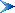## Aircraft Performance

 SECTION 1:  Properties of the Atmosphere  SECTION 2 :  Aircraft Weight, Geometry, Lift/Drag Coefficient and Engine Thrust Properties.  SECTION 3 :  Take-Off Performance.   SECTION 4 :  Climb Performance.   SECTION 5 :  Range & Endurance. The calculation of the performance of a fixed wing aircraft can be done using a set of simple force equilbrium equations.  In most flight conditions an aircraft will have force equilibrium in all three dimensions.  If a wind axis system is used with vertical being defined at right angles to the freestream velocity vector then the applied vertical forces will be a combination of lift, aircraft weight and ground support reaction forces. For the horizontal axis parallel to the stream direction the applied forces will consist of a combination of thrust, drag, weight components and ground friction effects. In the case of a turning aircraft the crosswind axis equilibrium also needs to be considered as there will be additional inertial forces generated by centripetal acceleration. When the aircraft is not in equilibrium then the resulting accelerations will cause dynamic motion which will need to be analysed by applying the equations of flight mechanics and control. In this chapter it is assumed that the aircraft is in equilibrium, or near equilibrium, so that the simpler aircraft performance equations can be applied. Calculations of take-off roll, field length, climb rate, climb gradient, ceiling, range, endurance, turn rate, descent angle and  landing roll will be shown using basic equilibrium equations.Table of Contents © AMME, University of Sydney, 1998-2006.